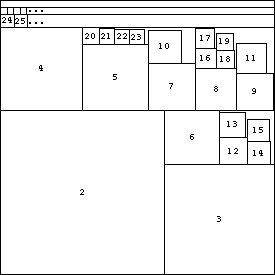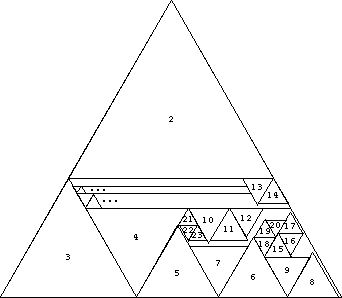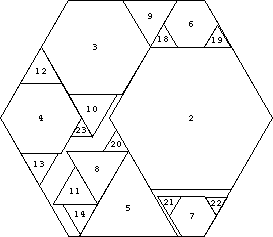# Harmonic Series of Polygons

In Unsolved Problems in Geometry, Leo Moser notes that 1/22 + 1/32 + 1/42 + . . . = pi2/6 - 1 < (5/6)2 and asks whether the squares of sides 1/2, 1/3, 1/4, . . . can be packed into a square of side 5/6 without overlap. The answer is yes, and one possible packing is shown below.For convenience, we have labelled a square n if it has side length 1/n . Similarly, we will call it square n in what follows. The positions of all the larger squares are shown. Squares 24, 25, . . . 47 are arranged in a row. Squares 48, 49, . . . 95 are arranged in a row above that, and so on.

The same sort of packing can be done for triangles.We can also do it for hexagons. The triangles stand for an infinite regress of hexagons. For example, the triangle labelled 5 contains hexagons (5), (15, 16, 17), (45, 46, 47, 48, 49, 50, 51, 52, 53), and so on.